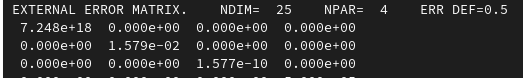# Pdf and function with different normalization (RooWrapperPdf)

Hi,

I created a personal pdf with RooWrapperPdf and my C++ Code.
In order to use the parameters in ongoing calculations, I wanted to know how I can get the right normalization factor for the function/parameters. I noticed when I use the same parameters on the same function, then the function doesn’t look the same as in the RooPlot frame (different normalization):

Blue fit is the pdf in RooPlot and red fit the same function as TF1.
My function is a simple gaussian with an amplitue parameter added:

[[nodiscard]] Double_t my_gauss(const Double_t x, const Double_t par) {
return par * exp(-(x-par)
(x-par)/(2
par*par));
}

I’ve read different approaches to this problem in the forum, but those haven’t worked for me. Does anyone have a “simple” solution to this problem that I haven’t discovered yet?